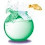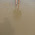## Tuesday, August 04, 2009

### What is the Value of F

What is the value of F in the following system?

A + B =  Z
Z + P = T
T + A = F
B + P + F = 30
A = 8

1.F equals 23.

If we label the equations in order (1) to (5) we have:
(1) & (2) => A + B + P = T
(4) => A + 30 - F = T
(3) => A + 30 - F = F - A
(5) => 46 = 2F => F = 23

2.F=23

Since A=8
8+B =Z from 1
subtitute 1 in 2
8 +B + P =T
subsitute above in 3
16 +B+P = F since A= 8 hence 2A=16
subs above in 4
we get
2B+2P+2A = 30
B+P+A=15
this gives
B+P=7
therefore
F=23

Visit: www.guessthelogo.blogspot.com

3.twenty three. hmmm the previous people found out how to find it alot less complicated then me. I found out the value of T when I substituted certain letters to get the equation: z-8+8+T+T-z=30. Then I got that T=15 and I almost published that before I realized that that was not what you wanted so I panicked thinking I had to somehow find another complicated equation, but just used the equation T+A=F and WUALLAAAA (an exclamation meaning it suddenly appeared :P)

4.Agreed. F=23. (and in case anyone wanted to check work, I'm pretty sure A=8, B=-8, P=15, and T=15 (Z is a free variable)... looking at Rich's answer, I think I made it more complicated than it should have been...wooo lin. alg.)

5.P=0
A=8
B=7
T=15
Z=15

F=23

6.7.Looks like there's consensus on this one. F = 23 is the answer.

8.What is -1/8f-3/4-1/4f=11?

Leave your answer or, if you want to post a question of your own, send me an e-mail. Look in the about section to find my e-mail address. If it's new, I'll post it soon.

Please don't leave spam or 'Awesome blog, come visit mine' messages. I'll delete them soon after.

Enter your Email and join hundreds of others who get their Question of the Day sent right to their mailbox

The Lamplight Manor Puzz 3-D